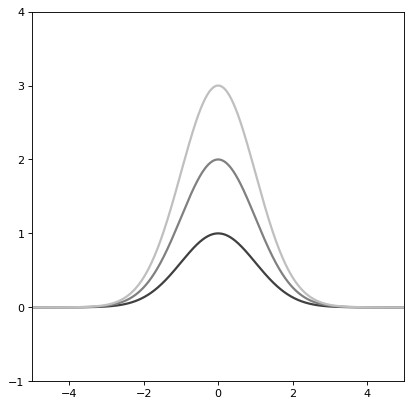# Gaussian1D¶

class astropy.modeling.functional_models.Gaussian1D(amplitude=1, mean=0, stddev=1, **kwargs)[source]

One dimensional Gaussian model.

Parameters
amplitudefloat

Amplitude of the Gaussian.

meanfloat

Mean of the Gaussian.

stddevfloat

Standard deviation of the Gaussian.

Other Parameters
fixeda dict, optional

A dictionary {parameter_name: boolean} of parameters to not be varied during fitting. True means the parameter is held fixed. Alternatively the fixed property of a parameter may be used.

tieddict, optional

A dictionary {parameter_name: callable} of parameters which are linked to some other parameter. The dictionary values are callables providing the linking relationship. Alternatively the tied property of a parameter may be used.

boundsdict, optional

A dictionary {parameter_name: value} of lower and upper bounds of parameters. Keys are parameter names. Values are a list or a tuple of length 2 giving the desired range for the parameter. Alternatively, the min and max properties of a parameter may be used.

eqconslist, optional

A list of functions of length n such that eqcons[j](x0,*args) == 0.0 in a successfully optimized problem.

ineqconslist, optional

A list of functions of length n such that ieqcons[j](x0,*args) >= 0.0 is a successfully optimized problem.

Notes

Model formula:

$f(x) = A e^{- \frac{\left(x - x_{0}\right)^{2}}{2 \sigma^{2}}}$

Examples

>>> from astropy.modeling import models
>>> def tie_center(model):
...         mean = 50 * model.stddev
...         return mean
>>> tied_parameters = {'mean': tie_center}


Specify that ‘mean’ is a tied parameter in one of two ways:

>>> g1 = models.Gaussian1D(amplitude=10, mean=5, stddev=.3,
...                             tied=tied_parameters)


or

>>> g1 = models.Gaussian1D(amplitude=10, mean=5, stddev=.3)
>>> g1.mean.tied
False
>>> g1.mean.tied = tie_center
>>> g1.mean.tied
<function tie_center at 0x...>


Fixed parameters:

>>> g1 = models.Gaussian1D(amplitude=10, mean=5, stddev=.3,
...                        fixed={'stddev': True})
>>> g1.stddev.fixed
True


or

>>> g1 = models.Gaussian1D(amplitude=10, mean=5, stddev=.3)
>>> g1.stddev.fixed
False
>>> g1.stddev.fixed = True
>>> g1.stddev.fixed
True

import numpy as np
import matplotlib.pyplot as plt

from astropy.modeling.models import Gaussian1D

plt.figure()
s1 = Gaussian1D()
r = np.arange(-5, 5, .01)

for factor in range(1, 4):
s1.amplitude = factor
plt.plot(r, s1(r), color=str(0.25 * factor), lw=2)

plt.axis([-5, 5, -1, 4])
plt.show()


()Attributes Summary

 amplitude fwhm Gaussian full width at half maximum. input_units This property is used to indicate what units or sets of units the evaluate method expects, and returns a dictionary mapping inputs to units (or None if any units are accepted). mean param_names stddev

Methods Summary

 evaluate(x, amplitude, mean, stddev) Gaussian1D model function. fit_deriv(x, amplitude, mean, stddev) Gaussian1D model function derivatives.

Attributes Documentation

amplitude
fwhm

Gaussian full width at half maximum.

input_units

This property is used to indicate what units or sets of units the evaluate method expects, and returns a dictionary mapping inputs to units (or None if any units are accepted).

Model sub-classes can also use function annotations in evaluate to indicate valid input units, in which case this property should not be overridden since it will return the input units based on the annotations.

mean
param_names = ('amplitude', 'mean', 'stddev')
stddev

Methods Documentation

static evaluate(x, amplitude, mean, stddev)[source]

Gaussian1D model function.

static fit_deriv(x, amplitude, mean, stddev)[source]

Gaussian1D model function derivatives.